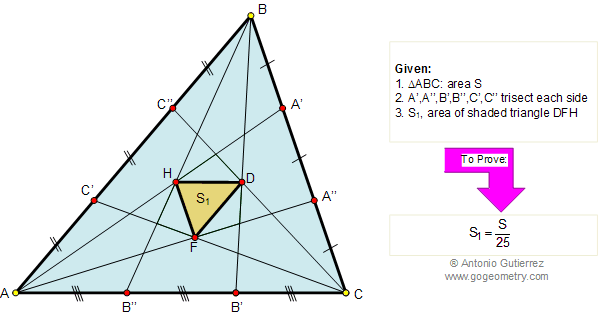Problem 124. Area of triangle, Similarity. Level: High School, SAT Prep, College
 In the figure below, prove that the area S1 of the central triangle region DFH determined by trisection of each side of a triangle ABC and connecting the corresponding points A', A", B', B", C', and C" with the opposite vertex is given by 1/25 the area of triangle ABC. View or post a solution.FACTS AND HINTS:

Geometry problem solving is one of the most challenging skills for students to learn. When a problem requires auxiliary construction, the difficulty of the problem increases drastically, perhaps because deciding which construction to make is an ill-structured problem. By “construction,” we mean adding geometric figures (points, lines, planes) to a problem figure that wasn’t mentioned as "given."

 Home | Search | Problems | Marion Walter Index | 121-130 | Segment Trisection | Email | By Antonio Gutierrez